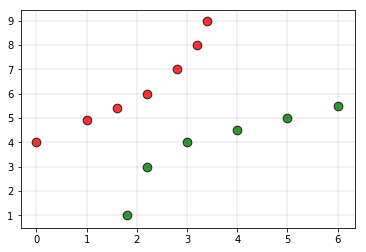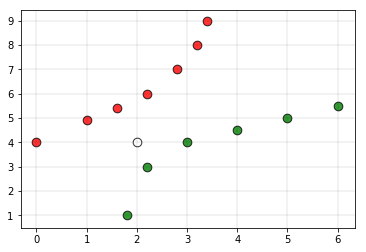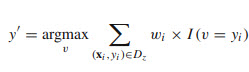Related Articles
Weighted K-NN
• Last Updated : 07 Apr, 2020

Weighted kNN is a modified version of k nearest neighbors. One of the many issues that affect the performance of the kNN algorithm is the choice of the hyperparameter k. If k is too small, the algorithm would be more sensitive to outliers. If k is too large, then the neighborhood may include too many points from other classes.
Another issue is the approach to combining the class labels. The simplest method is to take the majority vote, but this can be a problem if the nearest neighbors vary widely in their distance and the closest neighbors more reliably indicate the class of the object.

Intuition:
Consider the following training setThe red labels indicate the class 0 points and the green labels indicate class 1 points.
Consider the white point as the query point( the point whose class label has to be predicted)If we give the above dataset to a kNN based classifier, then the classifier would declare the query point to belong to the class 0. But in the plot, it is clear that the point is more closer to the class 1 points compared to the class 0 points. To overcome this disadvantage, weighted kNN is used. In weighted kNN, the nearest k points are given a weight using a function called as the kernel function. The intuition behind weighted kNN, is to give more weight to the points which are nearby and less weight to the points which are farther away. Any function can be used as a kernel function for the weighted knn classifier whose value decreases as the distance increases. The simple function which is used is the inverse distance function.

Algorithm:

• Let L = { ( xi , yi ) , i = 1, . . . ,n } be a training set of observations xi with given class yi and let x be a new observation(query point), whose class label y has to be predicted.
• Compute d(xi, x) for i = 1, . . . ,n , the distance between the query point and every other point in the training set.
• Select D’ ⊆ D, the set of k nearest training data points to the query points
• Predict the class of the query point, using distance-weighted voting. The v represents the class labels. Use the following formulaImplementation:
Consider 0 as the label for class 0 and 1 as the label for class 1. Below is the implementation of weighted-kNN algorithm.

## C/C++

 `// C++ program to implement the ``// weighted K nearest neighbour algorithm. ``#include  ``using` `namespace` `std; `` ` `struct` `Point ``{ ``    ``int` `val;     ``// Class of point ``    ``double` `x, y;     ``// Co-ordinate of point ``    ``double` `distance; ``// Distance from test point ``}; `` ` `// Used to sort an array of points by increasing ``// order of weighted distance ``bool` `comparison(Point a, Point b) ``{ ``    ``return` `(a.distance < b.distance); ``} `` ` `// This function finds classification of point p using ``// weighted k nearest neighbour algorithm. It assumes only  ``// two groups and returns 0 if p belongs to class 0, else ``// 1 (belongs to class 1). ``int` `weightedkNN(Point arr[], ``int` `n, ``int` `k, Point p) ``{ ``    ``// Fill weighted distances of all points from p ``    ``for` `(``int` `i = 0; i < n; i++) ``        ``arr[i].distance = ``            ``(``sqrt``((arr[i].x - p.x) * (arr[i].x - p.x) + ``                ``(arr[i].y - p.y) * (arr[i].y - p.y))); `` ` `    ``// Sort the Points by weighted distance from p ``    ``sort(arr, arr+n, comparison); `` ` `    ``// Now consider the first k elements and only ``    ``// two groups ``    ``double` `freq1 = 0;     ``// weighted sum of group 0 ``    ``double` `freq2 = 0;     ``// weighted sum of group 1 ``    ``for` `(``int` `i = 0; i < k; i++) ``    ``{ ``        ``if` `(arr[i].val == 0) ``            ``freq1 += ``double``(1/arr[i].distance); ``        ``else` `if` `(arr[i].val == 1) ``            ``freq2 += ``double``(1/arr[i].distance); ``    ``} ``    ``return` `(freq1 > freq2 ? 0 : 1); ``} `` ` `// Driver code ``int` `main() ``{ ``    ``int` `n = 13; ``// Number of data points ``    ``Point arr[n]; `` ` `    ``arr.x = 0; ``    ``arr.y = 4; ``    ``arr.val = 0; `` ` `    ``arr.x = 1; ``    ``arr.y = 4.9; ``    ``arr.val = 0; `` ` `    ``arr.x = 1.6; ``    ``arr.y = 5.4; ``    ``arr.val = 0; `` ` `    ``arr.x = 2.2; ``    ``arr.y = 6; ``    ``arr.val = 0; `` ` `    ``arr.x = 2.8; ``    ``arr.y = 7; ``    ``arr.val = 0; `` ` `    ``arr.x = 3.2; ``    ``arr.y = 8; ``    ``arr.val = 0; `` ` `    ``arr.x = 3.4; ``    ``arr.y = 9; ``    ``arr.val = 0; `` ` `    ``arr.x = 1.8; ``    ``arr.y = 1; ``    ``arr.val = 1; `` ` `    ``arr.x = 2.2; ``    ``arr.y = 3; ``    ``arr.val = 1; `` ` `    ``arr.x = 3; ``    ``arr.y = 4; ``    ``arr.val = 1; `` ` `    ``arr.x = 4; ``    ``arr.y = 4.5; ``    ``arr.val = 1; `` ` `    ``arr.x = 5; ``    ``arr.y = 5; ``    ``arr.val = 1; `` ` `    ``arr.x = 6; ``    ``arr.y = 5.5; ``    ``arr.val = 1; `` ` `    ``/*Testing Point*/``    ``Point p; ``    ``p.x = 2; ``    ``p.y = 4; `` ` `    ``// Parameter to decide the class of the query point ``    ``int` `k = 5; ``    ``printf` `(``"The value classified to query point"``            ``" is: %d.\n"``, weightedkNN(arr, n, k, p)); ``    ``return` `0; ``} `

## Python3

 `# Python3 program to implement the``# weighted K nearest neighbour algorithm. `` ` `import` `math `` ` `def` `weightedkNN(points,p,k``=``3``): ``    ``''' ``    ``This function finds classification of p using ``    ``weighted k nearest neighbour algorithm. It assumes only two ``    ``two classes and returns 0 if p belongs to class 0, else ``    ``1 (belongs to class 1). `` ` `    ``Parameters - ``        ``points : Dictionary of training points having two keys - 0 and 1 ``            ``Each key have a list of training data points belong to that `` ` `        ``p : A tuple ,test data point of form (x,y) `` ` `        ``k : number of nearest neighbour to consider, default is 3 ``    ``'''`` ` `    ``distance``=``[] ``    ``for` `group ``in` `points: ``        ``for` `feature ``in` `points[group]: `` ` `            ``#calculate the euclidean distance of p from training points ``            ``euclidean_distance ``=` `math.sqrt((feature[``0``]``-``p[``0``])``*``*``2` `+``(feature[``1``]``-``p[``1``])``*``*``2``) `` ` `            ``# Add a tuple of form (distance,group) in the distance list ``            ``distance.append((euclidean_distance,group)) `` ` `    ``# sort the distance list in ascending order ``    ``# and select first k distances ``    ``distance ``=` `sorted``(distance)[:k] `` ` `    ``freq1 ``=` `0` `# weighted sum of group 0 ``    ``freq2 ``=` `0` `# weighted sum of group 1 `` ` `    ``for` `d ``in` `distance:``        ``if` `d[``1``] ``=``=` `0``:``            ``freq1 ``+``=` `(``1` `/` `d[``0``])``             ` `        ``elif` `d[``1``] ``=``=` `1``: ``            ``freq2 ``+``=` `(``1` `/``d[``0``])``             ` ` ` `    ``return` `0` `if` `freq1>freq2 ``else` `1`` ` `# Driver function ``def` `main(): `` ` `    ``# Dictionary of training points having two keys - 0 and 1 ``    ``# key 0 have points belong to class 0 ``    ``# key 1 have points belong to class 1 `` ` `    ``points ``=` `{``0``:[(``0``, ``4``),(``1``, ``4.9``),(``1.6``, ``5.4``),(``2.2``, ``6``),(``2.8``, ``7``),(``3.2``, ``8``),(``3.4``, ``9``)], ``            ``1``:[(``1.8``, ``1``),(``2.2``, ``3``),(``3``, ``4``),(``4``, ``4.5``),(``5``, ``5``),(``6``, ``5.5``)]} `` ` `    ``# query point p(x,y) ``    ``p ``=` `(``2``, ``4``) `` ` `    ``# Number of neighbours ``    ``k ``=` `5`` ` `    ``print``(``"The value classified to query point is: {}"``.``format``(weightedkNN(points,p,k))) `` ` `if` `__name__ ``=``=` `'__main__'``: ``    ``main() `
Output:
```The value classified to query point is: 1
```
Want to learn from the best curated videos and practice problems, check out the C++ Foundation Course for Basic to Advanced C++ and C++ STL Course for foundation plus STL.  To complete your preparation from learning a language to DS Algo and many more,  please refer Complete Interview Preparation Course.

My Personal Notes arrow_drop_up